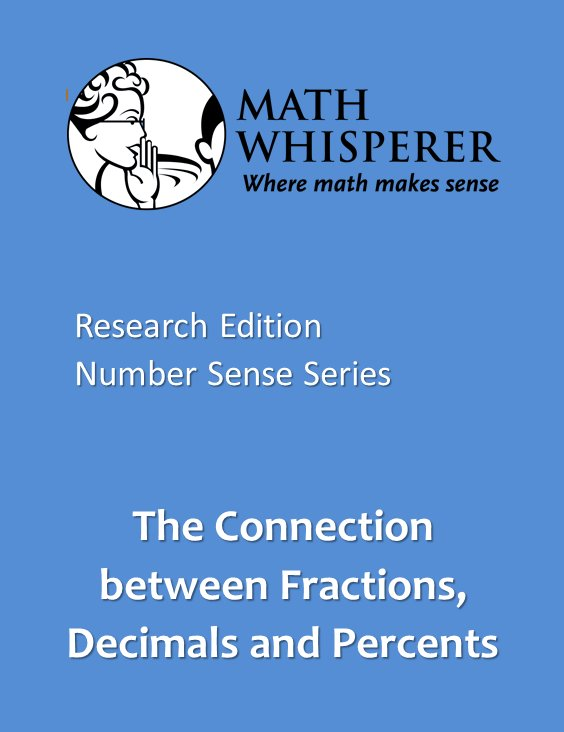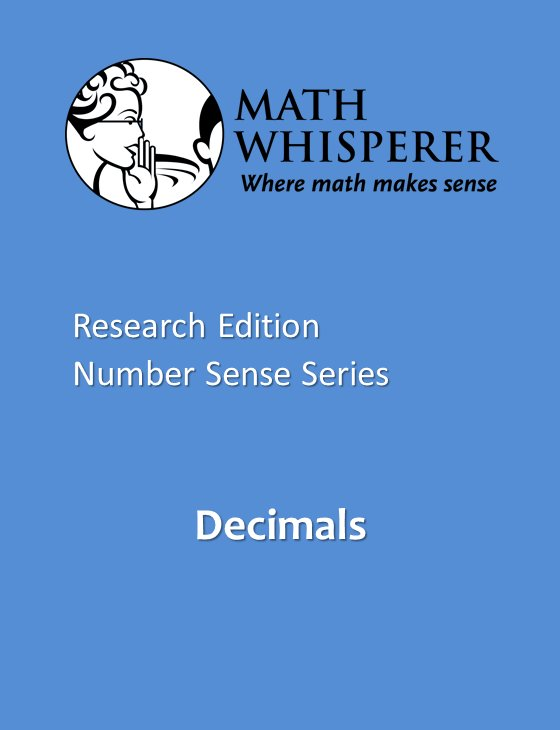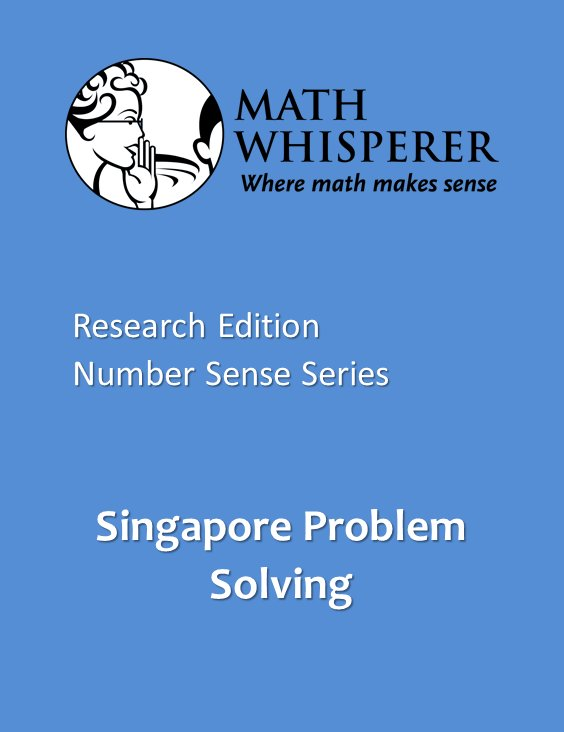Select Page

# Number Sense Series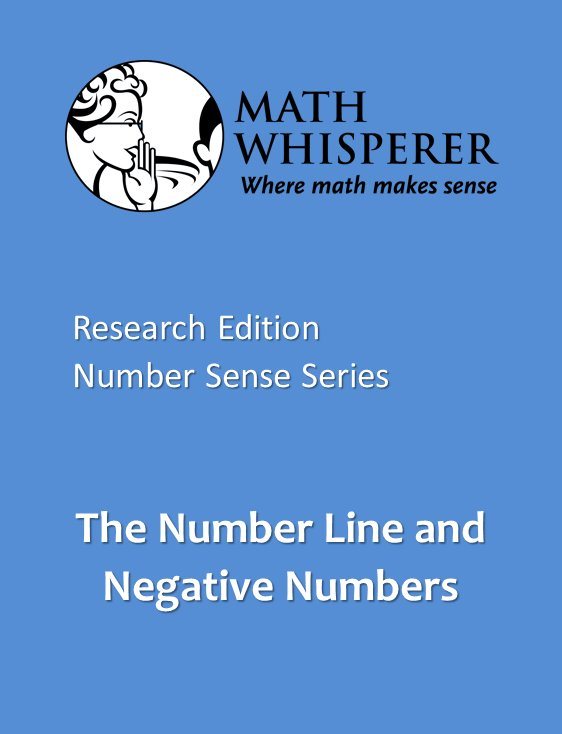Math Whisperer materials are dedicated to each person who wants to be successful in math, including those who have struggled in the past. Our goal for our students is that they know the math they need to lead the lives they want.

Grade 6 and above: Understanding negative numbers.
Grade 8 and above: Calculating with positive and negative numbers using a powerful model.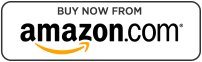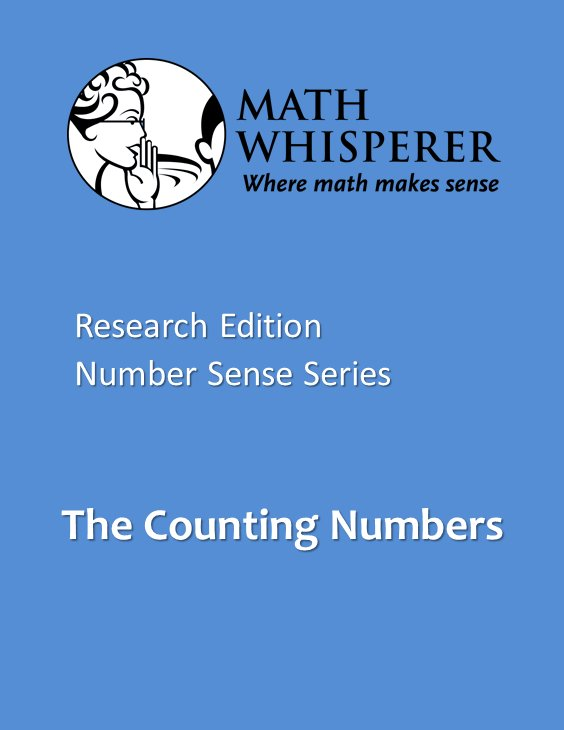Math Whisperer materials are dedicated to each person who wants to be successful in math, including those who have struggled in the past. Our goal for our students is that they know the math they need to lead the lives they want.

Grades 2 and above: Our numbers are based on “tens.” While this seems obvious to most adults, many students do not actually understand this fundamental way our numbers are constructed. This book uses a popsicle stick model and game to help students understand numbers through the hundreds.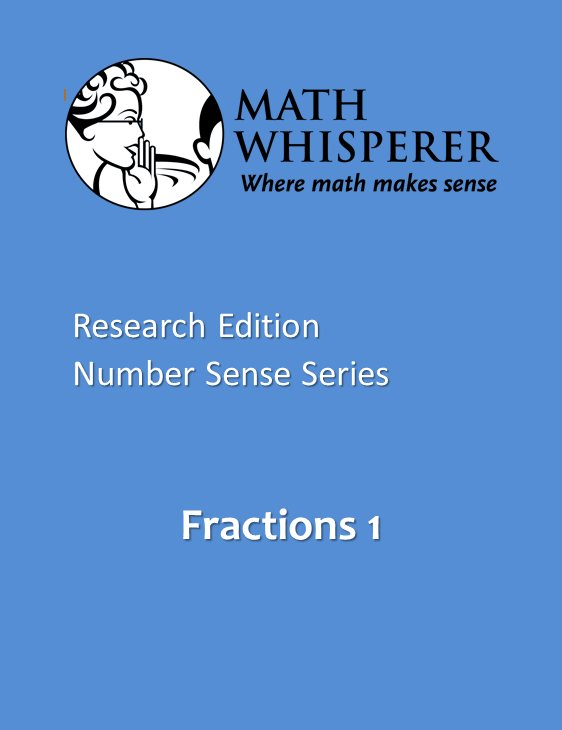Fractions are critical to success in algebra. In this book, fractions will make sense to you and you will never have to learn them again.

Grades 6 and above:  Fractions are important in their own right, and essential for Algebra.   To be successful in Algebra, students must understand what fractions are all about, and how to multiply them.  In this book, multiplying fractions and reducing fractions actually make sense, so that students can understand and remember how to do those problems.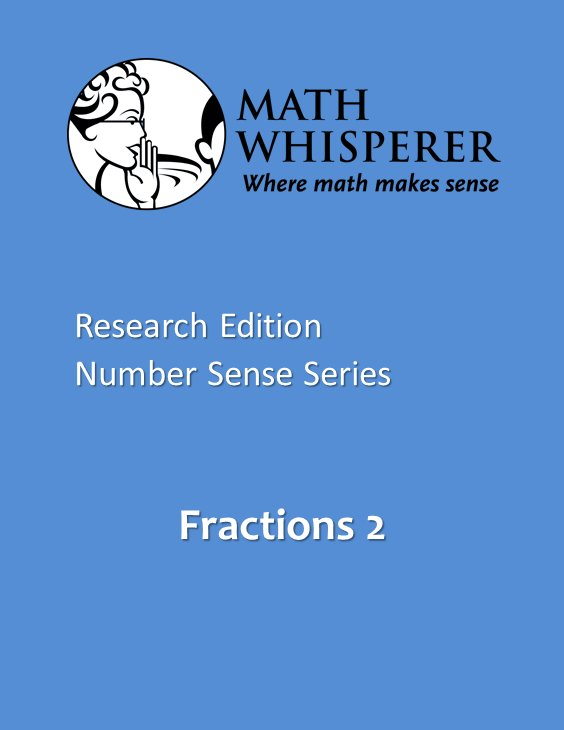Fractions are critical to success in algebra. In this book, fractions will make sense to you and you will never have to learn them again.

Grades 7 and above:  Fractions are important in their own right, and essential for Algebra.  To be successful in Algebra, students must be able to subtract, add and divide fractions.  In this book, those fraction operations actually make sense, so that students can understand and remember them.## Coming soon